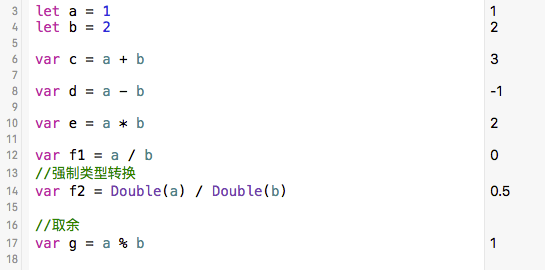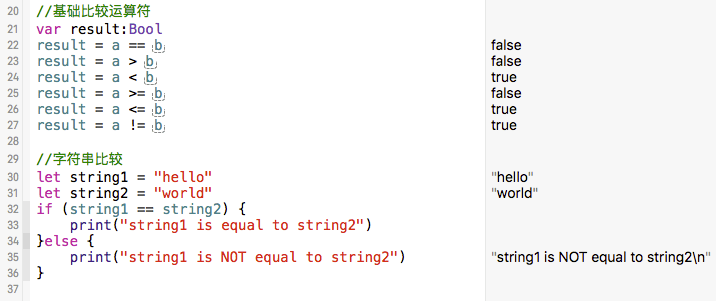# 基本运算符

``````let a = 1
let b = 2

var c = a + b

var d = a - b

var e = a * b

var f1 = a / b
//强制类型转换
var f2 = Double(a) / Double(b)

//取余
var g = a % b

``````# 比较运算符

``````//基础比较运算符
var result:Bool
result = a == b
result = a > b
result = a < b
result = a >= b
result = a <= b
result = a != b

//字符串比较
let string1 = "hello"
let string2 = "world"
if (string1 == string2) {
print("string1 is equal to string2")
}else {
print("string1 is NOT equal to string2")
}
``````# 代码下载

https://github.com/99ios/SwiftBasic